40m QIL Cryo_Lab CTN SUS_Lab TCS_Lab OMC_Lab CRIME_Lab FEA ENG_Labs OptContFac Mariner WBEEShop
 COMSOL elog Not logged inSat Jun 28 21:59:11 2014, Sam Moore, Optics, General, Difficulty with the COMSOL stationary module; Test CasesSun Jun 29 15:37:18 2014, Sam Moore, Optics, General, Difficulty with the COMSOL stationary module; Test CasesSun Jun 29 20:25:44 2014, Koji, Optics, General, Difficulty with the COMSOL stationary module; Test Cases
Message ID: 90     Entry time: Sun Jun 29 20:25:44 2014     In reply to: 88
 Author: Koji Type: Optics Category: General Subject: Difficulty with the COMSOL stationary module; Test Cases

Consider a bar with the length of L.

Let's say there is no body heat applied, but the temperature of the bar at x=L is kept at T=0
and at x=0 is kept at T=T0 Exp[I w t].

The equation for the bar is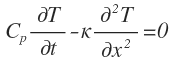...(1)

Consider the solution with the form of T(x, t) = T(x) T0 Exp(I w t), where T(x) is the position dependent transfer function.
T(x) is a complex function.

Eq.1 is modified with T(x) as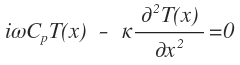With the boundary condition of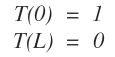This can be analytically solved in the following form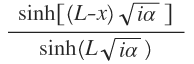where alpha is defined by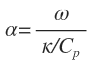So kappa/Cp is the characteristic (angular) frequency of the system.
Here is the example plot for L=1 and alpha = 1 (red), 10 (yellow green), 100 (turquoise), 1000 (blue)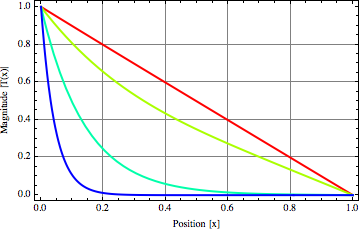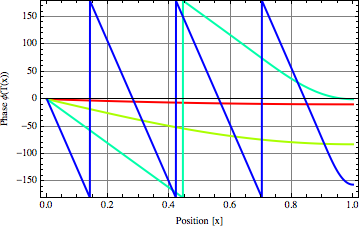If the oscillation is slow enough, the temperature decay length is longer than the bar length and thus the temperature is linear to the position.
If the oscillation is fast, the decay length is significantly shorter  than the bar length and the temperature dependence on the position is exponential.

Now what we need is to solve this in COMSOL

ELOG V3.1.3-